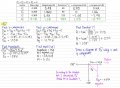# Solve for a vector needed to cancel out given sets of forces

#### asilvester635

Joined Jan 26, 2017
73
For this assignment, I am given sets of forces and asked to solve for the force(s) needed to cancel them out. In this case, I am asked to solve for the last vector, which is vector D. I've successfully found its x and y component, magnitude, and mass (kg). I've also checked if all of their combined x and y components added up to zero by adding the x and y components for all vectors A, B, C, and D. What I don't understand is the answer I got for the direction of vector D. When I drew a diagram for vector D, it's located in quadrant 4, since x-component is 1.23 N, and y-component is -0.37 N. The angle I got was 253 degrees, which means that the vectors should be in quadrant 3, but according to my x and y component, it's pointing in quadrant 4. Did I make a mistake when calculating the direction of vector D? Below is my work.#### RBR1317

Joined Nov 13, 2010
621
In the old days we would plot the vectors on polar graph paper, then add the vectors with a bow compass and triangle.